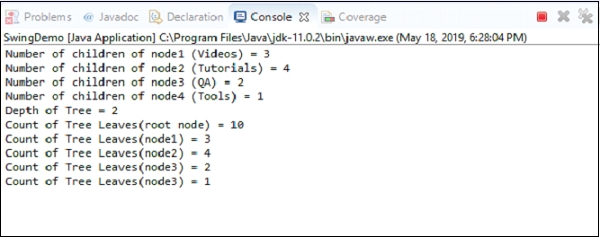# How to get the leaves of nodes in a JTree with Java?

Java 8Object Oriented ProgrammingProgramming

To get the leaves of nodes, use the getLeafCount() method.

Let’s say you want the leaves of the entire tree, then use the root node, Let’s say “node” is our root node −

node.getLeafCount()

Now, let’s say we want to get the leaves of a node, which is not a root node, therefore set the node. Here, node1 is not a root node −

node1.getLeafCount()

The following is an example to get the leaves of nodes −

## Example

package my;
import javax.swing.JFrame;
import javax.swing.JTree;
import javax.swing.tree.DefaultMutableTreeNode;
public class SwingDemo {
public static void main(String[] args) throws Exception {
JFrame frame = new JFrame("Demo");
DefaultMutableTreeNode node = new DefaultMutableTreeNode("Website");
DefaultMutableTreeNode node1 = new DefaultMutableTreeNode("Videos");
DefaultMutableTreeNode node2 = new DefaultMutableTreeNode("Tutorials");
DefaultMutableTreeNode node3 = new DefaultMutableTreeNode("QA");
DefaultMutableTreeNode node4 = new DefaultMutableTreeNode("Tools");
DefaultMutableTreeNode one = new DefaultMutableTreeNode("PHP Videos");
DefaultMutableTreeNode two = new DefaultMutableTreeNode("HTML5 Videos");
DefaultMutableTreeNode three = new DefaultMutableTreeNode("Blockchain Videos");
DefaultMutableTreeNode four = new DefaultMutableTreeNode("Java");
DefaultMutableTreeNode five = new DefaultMutableTreeNode("DBMS");
DefaultMutableTreeNode six = new DefaultMutableTreeNode("CSS");
DefaultMutableTreeNode seven = new DefaultMutableTreeNode("MongoDB");
DefaultMutableTreeNode eight = new DefaultMutableTreeNode("Python QA");
DefaultMutableTreeNode nine = new DefaultMutableTreeNode("jQuery QA");
DefaultMutableTreeNode ten = new DefaultMutableTreeNode("Photo Editing Tool");
JTree tree = new JTree(node);
for (int i = 0; i < tree.getRowCount(); i++) {
tree.expandRow(i);
}
tree.putClientProperty("JTree.lineStyle", "Angled");
System.out.println("Number of children of node1 (Videos) = " + node1.getChildCount());
System.out.println("Number of children of node2 (Tutorials) = " + node2.getChildCount());
System.out.println("Number of children of node3 (QA) = " + node3.getChildCount());
System.out.println("Number of children of node4 (Tools) = " + node4.getChildCount());
System.out.println("Depth of Tree = " + node.getDepth());
System.out.println("Count of Tree Leaves(root node) = " + node.getLeafCount());
System.out.println("Count of Tree Leaves(node1) = " + node1.getLeafCount());
System.out.println("Count of Tree Leaves(node2) = " + node2.getLeafCount());
System.out.println("Count of Tree Leaves(node3) = " + node3.getLeafCount());
System.out.println("Count of Tree Leaves(node3) = " + node4.getLeafCount());
tree.setRowHeight(25);
frame.setSize(600,450);
frame.setVisible(true);
}
}

## OutputFollowing is our JTree −